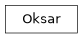# geoana.earthquake.oksar.Oksar#class geoana.earthquake.oksar.Oksar(O, U, V, center, depth_top, depth_bottom=10000.0, strike=0.0, dip=45.0, rake=90, slip=0.5, length=10000.0, beta=30000000000.0, mu=30000000000.0, shape=(300, 300))#

Attributes

 `O` Origin of the simulation domain. `U` U direction of the simulation domain `V` V direction of the simulation domain `beta` Beta parameter `center` Earthquake epicenter (bottom N, left E). `depth_bottom` Depth to the bottom of the fault (m) `depth_top` Depth to the top of the fault (m) `dip` Strike angle (0 to 90) in degrees `displacement_vector` Compute displacement vector `length` Length `mu` Mu parameter `rake` Rake angle (-180 to 180) `shape` Shape of the interferogram defined by number of pixels in the North and Easting directions, respectively; i.e. (n_pix_N, n_pix_E). `simulation_grid` Compute simulation grid `slip` Slip `strike` Strike angle (0 to 360) in degrees

Methods

 `plot_displacement`([eq, ax, wrap, mask_opacity]) Plot displacement/